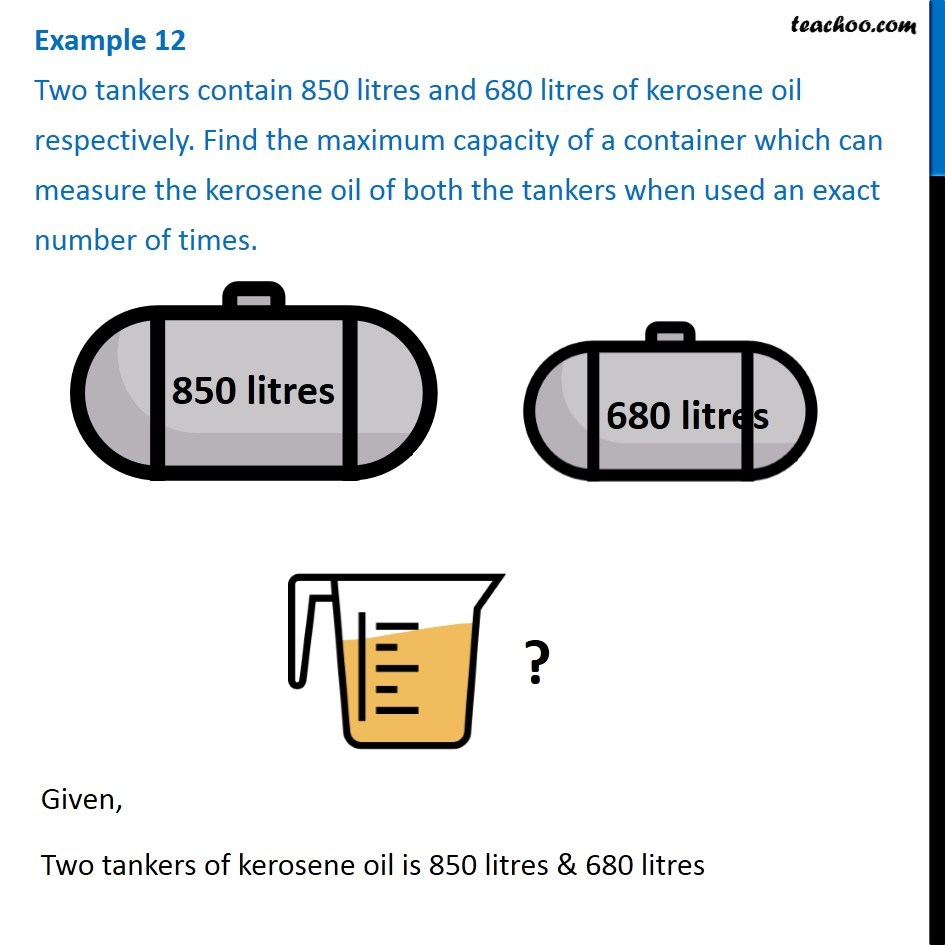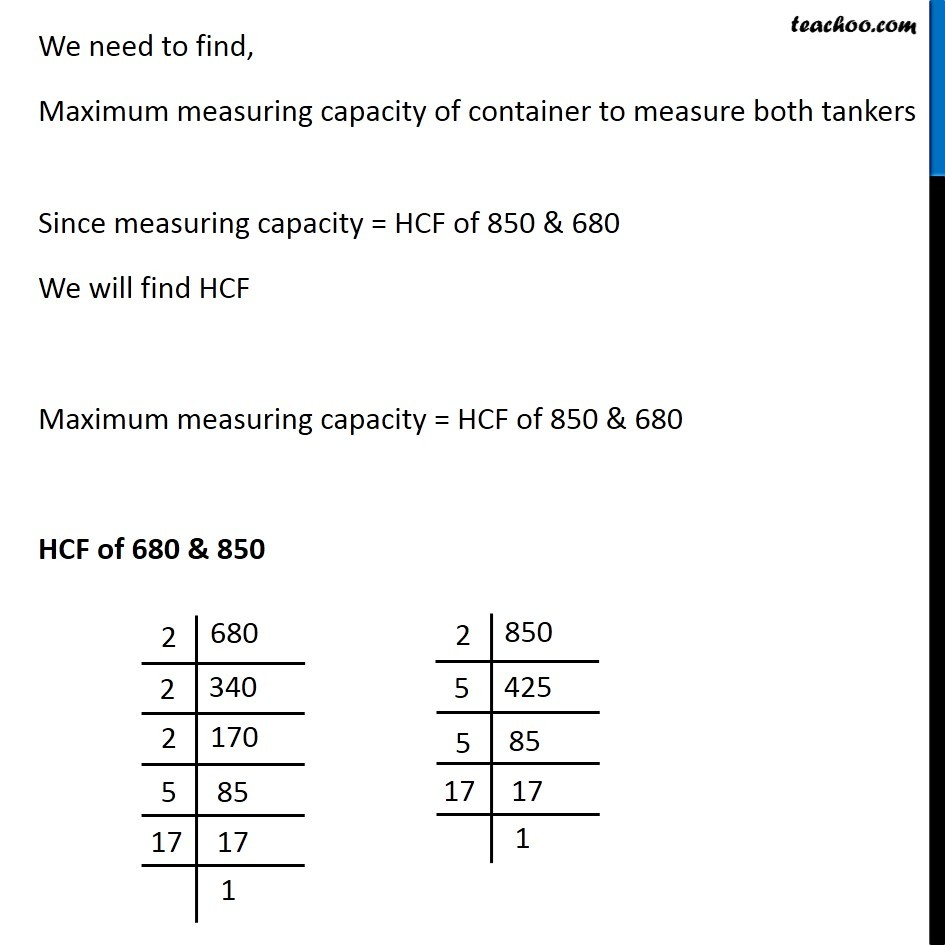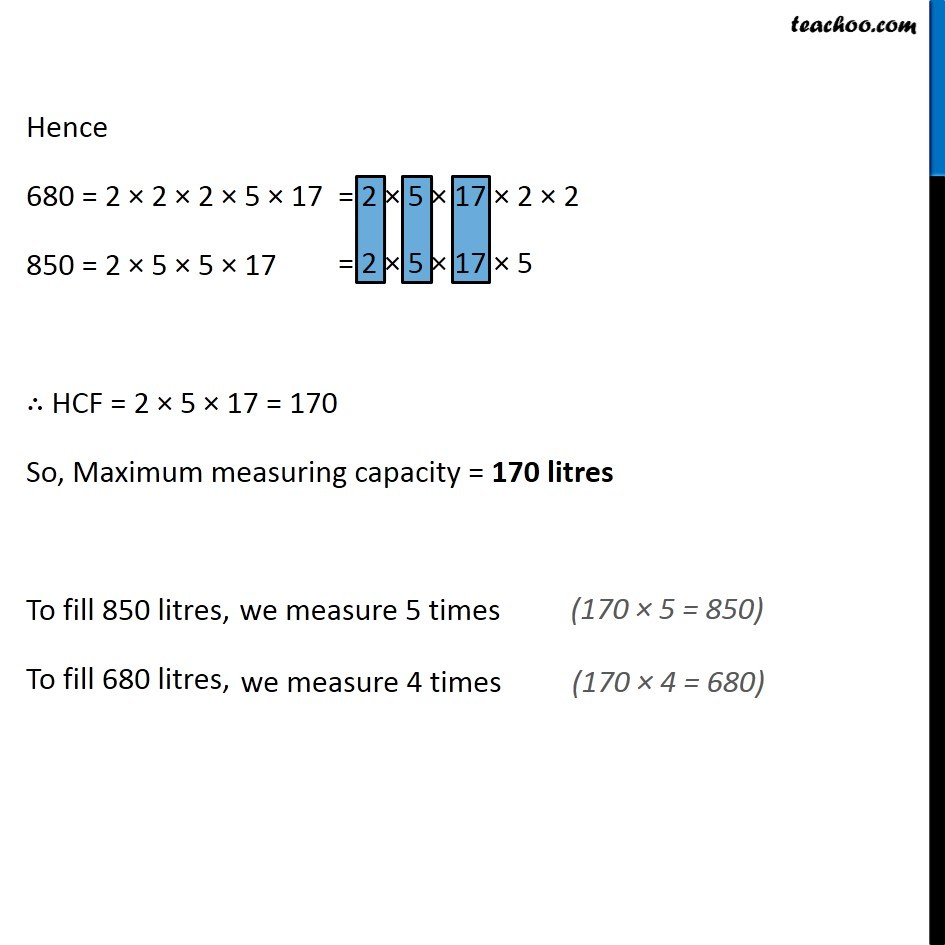1. Chapter 3 Class 6 Playing with Numbers
2. Concept wise
3. Statement questions on HCF

Transcript

Example 12 Two tankers contain 850 litres and 680 litres of kerosene oil respectively. Find the maximum capacity of a container which can measure the kerosene oil of both the tankers when used an exact number of times. Given, Two tankers of kerosene oil is 850 litres & 680 litres We need to find, Maximum measuring capacity of container to measure both tankers Since measuring capacity = HCF of 850 & 680 We will find HCF Maximum measuring capacity = HCF of 850 & 680 HCF of 680 & 850 Hence 680 = 2 × 2 × 2 × 5 × 17 850 = 2 × 5 × 5 × 17 ∴ HCF = 2 × 5 × 17 = 170 So, Maximum measuring capacity = 170 litres To fill 850 litres, To fill 680 litres,

Statement questions on HCF